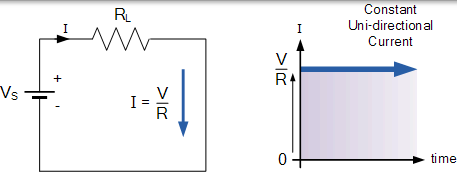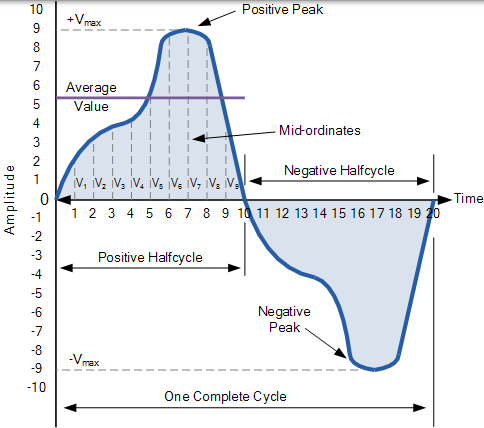# 交流波形与交流电路理论### 直流电路和波形AC 给出它对交流电的完整描述，通常指的是时变波形，其中最常见的被称为正弦波。到目前为止，正弦波是电气工程中最重要的交流波形之一。

### 交流波形特性

• 周期 T 是波形从开始到结束重复自身的时间长度（以秒为单位）。这也可以称为正弦波波形的周期时间，或方波的脉冲宽度。
• 频率 ƒ 是波形在一秒钟内重复出现的次数。频率是周期的倒数，（ ƒ= 1 / T），频率单位是赫兹，（Hz）。
• 幅度 A 是以伏特或安培为单位测量的信号波形的幅度或强度。

### 周期波形的类型AC 波形从其正半部分到其负半部分再完成一个完整模式所需的时间称为周期，一个完整周期包含正半周期和负半周期。波形完成一个完整周期所用的时间称为波形的周期时间，并给出符号 T

## AC 波形示例 No1

1. 50Hz 波形的周期时间是多少？
2. 周期时间为 10ms 的交流波形的频率是多少？

1. $$T =\frac {1}{f} = \frac {1}{50}=0.02\textrm{ s }\quad or \quad 20\textrm{ ms }$$

2. $$f = \frac {1}{T} = \frac {1}{10ms} = 100\textrm{ Hz }$$

### 频率前缀的定义

k kHz 1 ms
M 百万 MHz 1 us
G 十亿 GHz 1 ns
T 万亿 THz 1 ps

## 交流波形的平均值

### 非正弦波形的平均值## AC 波形的 RMS 值

Vrms 或 Irms 由正弦波的所有中间值的平方之和的平均值的平方根来得到。任何 AC 波形的 RMS 值可以从以下修改的平均值公式中找到。

### AC 波形的 RMS 值

$$V _ { R M S } = \sqrt { \frac { V _ { 1 } ^ { 2 } + V _ { 2 } ^ { 2 } + V _ { 3 } ^ { 2 } + V _ { 4 } ^ { 2 } + \ldots + V _ { n } ^ { 2 } } { n } }$$

## 形状因子和波峰因数

$$\text { Form Factor } = \frac { \text { RMS value } } { \text { Average value } } = \frac { 0.707 \times V \max } { 0.637 \times V \max }$$

$$\text { Crest Factor } = \frac { \text { Peak value } } { \text { RMS value } } = \frac { V \max } { 0.707 \times V \max }$$

## AC 波形示例 No2

6 安培的正弦交流电流经 40Ω 的电阻。计算电源的平均电压和峰值电压。

RMS 电压值计算如下： $$V _ { \mathrm { RMS } } = \mathrm { I } \times \mathrm { R } = 6 \times 40 = 240 \mathrm { V }$$

$${ \text { Form Factor } = \frac { V _ { \text { RM } } } { V _ { \text { average } } } }$$

$${ \therefore \text { Vaverage } = \frac { V _ { \text { RM } } } { \text { Form Factor } } = \frac { 240 } { 1.11 } = 216.2 \text { volts } }$$

$$\text { Peak Voltage } = \mathrm { R.M.S. } \times 1.414$$

$$\therefore 240 \times 1.414 = 339.4 \mathrm { volts }$$

### 正弦波形转换表

2 (√2)2 峰-峰值

0.707 1/(√2) RMS
0.637 2/π 平均

RMS 1.414 √2
RMS 0.901 (2√2)/π 平均